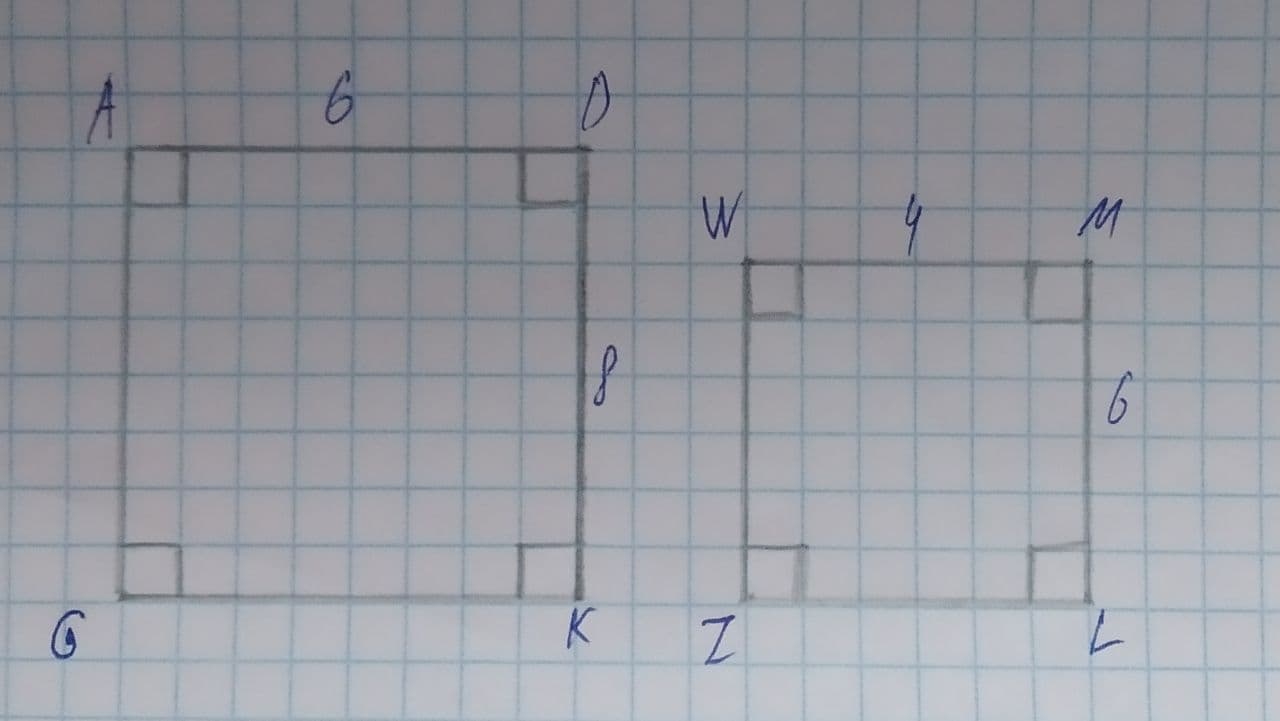# Determine whatever each pair if figures is similar. If so, write the similarity statement and scale factor. If not, explain your reasoning.Reeves 2021-08-05 Answered
Determine whatever each pair if figures is similar. If so, write the similarity statement and scale factor.You can still ask an expert for help

• Questions are typically answered in as fast as 30 minutes

Solve your problem for the price of one coffee

• Math expert for every subject
• Pay only if we can solve itNathalie Redfern

$\mathrm{\angle }A=\mathrm{\angle }W$
$\mathrm{\angle }Q=\mathrm{\angle }Z$
$\mathrm{\angle }D=\mathrm{\angle }M$
$\mathrm{\angle }K=\mathrm{\angle }L$
$\frac{AD}{WM}=\frac{6}{4}=1.5$
$\frac{DK}{ML}=\frac{8}{6}=\frac{4}{3}$
$\frac{AD}{WM}\ne \frac{DK}{ML}$
So, figure is no similar.